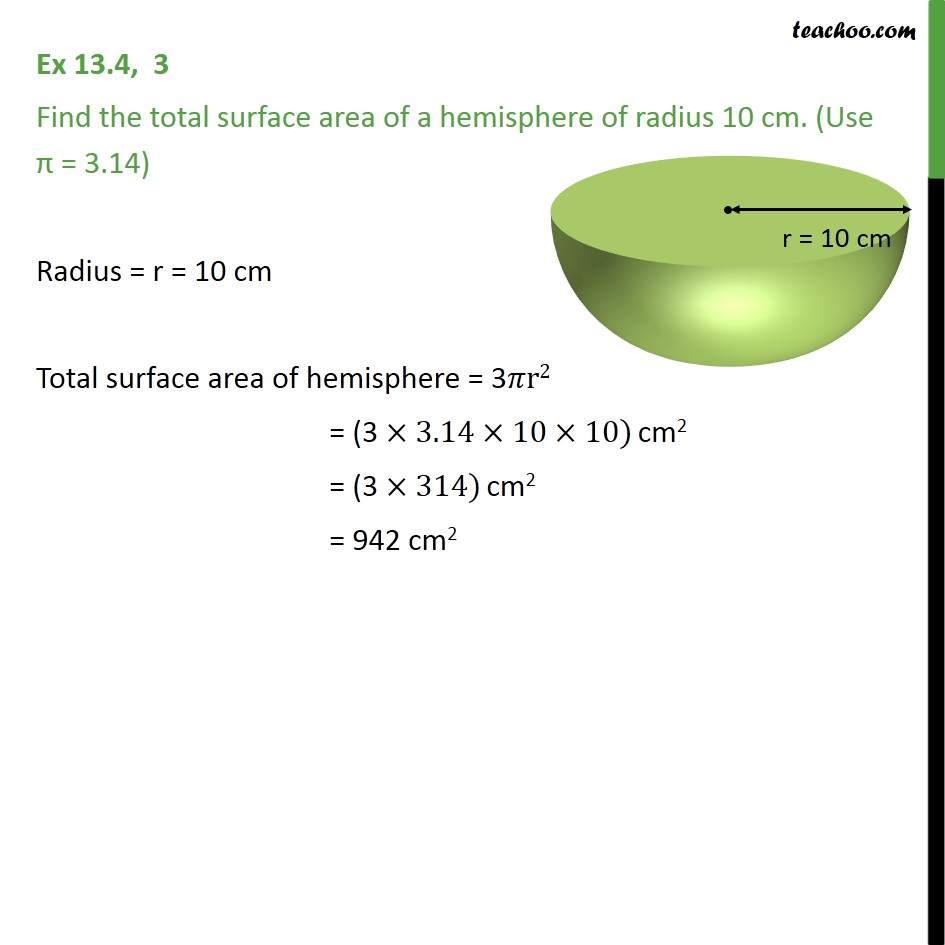Subscribe to our Youtube Channel - https://you.tube/teachoo

1. Chapter 13 Class 9 Surface Areas and Volumes
2. Serial order wise
3. Ex 13.4

Transcript

Ex 13.4, 3 Find the total surface area of a hemisphere of radius 10 cm. (Use = 3.14) Radius = r = 10 cm Total surface area of hemisphere = 3 r2 = (3 3.14 10 10) cm2 = (3 314) cm2 = 942 cm2

Ex 13.4